# Sheet Resistance

New for April 2010! This page gathers together a several others so they are easier to find. Most of the material was recently split off from our "conductivity" page. Yes, we know, it still needs some consolidation, come back tomorrow!

### DC sheet resistance

The "DC" explanation of sheet resistance ignores skin depth. For resistor calculations, 99% of the time this is a reasonable approximation. For attenuation in metal traces at microwave frequencies, this assumption will lead you astray, and you need to read our content on RF sheet resistance.

Bulk resistivity is a the property which is independent of frequency and geometry. In microwaves, often we are dealing with thin films of conductors, which have been applied at a controlled thickness. A more convenient property to deal with in this case is sheet resistance. The sheet resistance of a metal film is often expressed in ohms/square. What's a square? Exactly that. Who's on first? I Don't Know's on second.

Recall the equation for calculating resistance from bulk resistivity: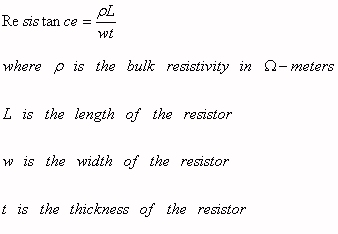If you consider the quantity L/w, it is unitless. It can be considered as a measure of how many squares of area your conductor or resistor has. For example, a thin-film resistor with length 30 mils and width 10 mils is three squares. A smaller resistor of 3 microns length and 1 microns width also has three squares (thanks Jack!) If they both have the same thickness and bulk resistivity, they both have the same value in ohms. They will have far different power ratings, and the smaller resistor will have a higher usable frequency response. Be careful not to mix up length and width, a resistor with 10 microns length and 30 microns width measures 1/3 square, not three squares!

Sheet resistance, Rsh, is equal to bulk resistivity divided by thickness. It can be used to conveniently calculate resistance values from number of squares, as follows:As in all engineering, you will need to keep units consistent in order to make the calculation correctly (if rho is in ohm centimeters, the thickness must also be in centimeters). One last thing to consider: sheet conductivity is the inverse of sheet resistivity. When is sheet conductivity useful? When you have more than one metal layer. The sheet conductivities of the layers can be added, because the conduction paths are in parallel.

The concept of sheet resistance is critical to an understanding of thin film resistors. The equation for resistance, based on bulk resistivity, is:

R=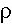L/Wt

where R is the resistance in ohmsis the bulk resistivity in micro-ohm centimeters

L is the length of the resistor in centimeters

W is the width of the resister in centimeters

and t is the thickness of the resistor in centimeters

In practice, you will have to convert units that you are given until they are all consistent with each other, but bulk resistivity is often expressed in micro-ohm centimeters.

For convenience, the quantity/t is used to express "sheet resistance", the units are ohms/square. For a given thin-film network (or MMIC), the sheet resistance should remain constant for all resistors, because the resistor material was uniformly deposited across the circuit. We can rewrite the equation this way:

R=L/Wt=(/t)x(L/W)=Rsheet x # squares

The quantity L/W is the number of "squares" that a resistor has, and total resistance is proportional to number of squares. In the figure below, we see a resistor with six squares (the light blue represents resistor material). If the sheet resistivity of the thin-film resistor is 50 ohms/square, we are looking at a 300 ohm resistor.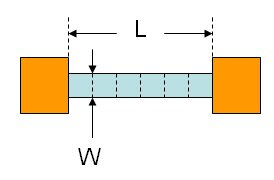Note that squares have no units. So you can measure length and width in microns, centimeters, mils, etc., and then determine the number of squares by dividing length by width. Also note that there are an infinite number of solutions to achieving the same ohm value for a thin-film resistor. A 1 mil x 1 mil resistor will have the same resistance as a 1 inch x 1 inch resistor. However, the one-mil-square resistor will have better high-frequency performance (because it is smaller), while the one-inch-square resistor will have a much higher power handling capability (because it spreads heat out more). These two resistors should have the same resistance value, even though they are of different size: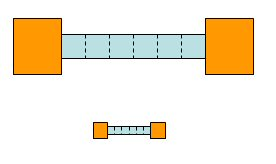Some other things to consider when designing resistors are that because of skin depth, the RF sheet resistance might be higher than the DC sheet resistance (but usually it is very close), and the tolerance of the resistor due to edge definition and other things might force you to laser trim it.

A mistake to avoid is to count the number of squares as W/L instead of L/W. The resistor below might look like it has five squares, when in reality it has only 0.2 squares: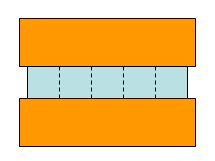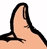Microwaves101 Rule of Thumb

When you are counting the number of squares in a meandering resistor, the squares at each bend should be counted as 1/2 square. In the figure below, if you count up all of the resistor squares, you'll get 43. But when you measure the resistor, it will behave like it has only 40 squares. That is because you need to reduce the corner squares by 50%.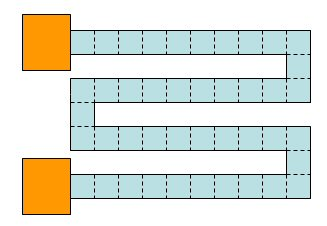Remember, the resistance calculated this way does not account for skin depth effects. It is accurate if your conductor thickness is small compared to a skin depth.

### RF sheet resistance

Because of skin depth, the effective sheet resistance changes with frequency, but only in a meaningful amount if the resistor's thickness is a less than a few skin depths. The content links below will help you understand the increase in sheet resistance due to the skin effect.

RF sheet resistance (separate page)

RF sheet resistance examples (separate page)

Author : Unknown Editor